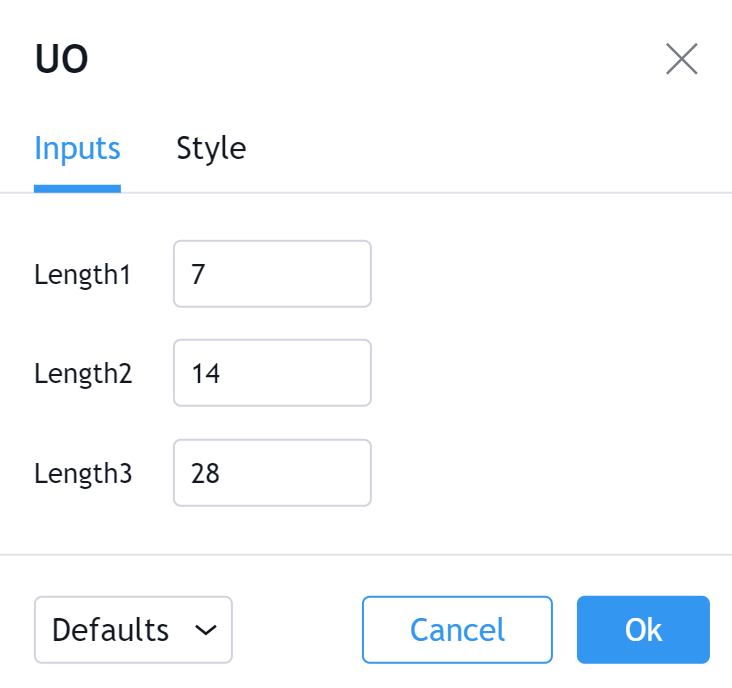# Ultimate Oscillator (UO)

#### Definition

The Ultimate Oscillator indicator (UO) indicator is a technical analysis tool used to measure momentum across three varying timeframes. The problem with many momentum oscillators is that after a rapid advance or decline in price, they can form false divergence trading signals. For example, after a rapid rise in price, a bearish divergence signal may present itself, however price continues to rise. The ultimate Oscillator attempts to correct this by using multiple timeframes in its calculation as opposed to just one timeframe which is what is used in most other momentum oscillators.

#### History

Larry Williams developed the Ultimate Oscillator (UO) in 1976. The indicator was then featured in Stocks & Commodities Magazine in 1985.

#### Calculation

There are three steps to calculating the Ultimate Oscillator. This example uses 7, 14, 28 parameters:
1. Before calculating the Ultimate Oscillator, two variable need to be defined; Buying Pressure and True Range.

```Buying Pressure (BP) = Close - Minimum (Lowest between Current Low or Previous Close)
True Range (TR) = Maximum (Highest between Current High or Previous Close) - Minimum (Lowest between Current Low or Previous Close)```

2. The Ultimate Oscillator then uses these figures over three time periods:

```Average7 = (7 Period BP Sum) / (7 Period TR Sum)
Average14 = (14 Period BP Sum) / (14 Period TR Sum)
Average28 = (28 Period BP Sum) / (28 Period TR Sum)```

3. The final Ultimate Oscillator calculations can now be made:

`UO = 100 x [(4 x Average7)+(2 x Average14)+Average28]/(4+2+1)`

#### The basics

A straightforward simplification of the Ultimate Oscillator indicator (UO) is that it is a way to measure buying pressure. When buying pressure is strong, the UO rises and when buying pressure is weak, the UO falls. The calculation for the UO takes into account three separate timeframes. Those timeframes are then weighted accordingly. This is the most important feature of the UO because it gives the most weight to the shortest time frame, yet it still factors in the longest timeframe. The goal of this is to avoid false divergences.

A technical analyst may find that they need to adjust the indicator's parameters. Overbought and oversold readings are essential to trading signals generated by the Ultimate Oscillator. Sometimes, financial instruments do not have enough price movement or volatility to generate overbought and oversold signals. Other times, instruments with a lot of volatility produce overbought and oversold readings too often. Historical analysis and research can assist in finding appropriate indicator parameters.

#### What to look for

The primary trading signals generated by the Ultimate Oscillator are bullish and bearish divergences. There are three conditions to both of these set ups.

##### Bullish UO Divergence
1. Bullish Divergence forms meaning price forms a lower low while UO makes a higher low.
2. The low of the Divergence should be below 30.
3. UO breaks above the high of the Divergence.

##### Bearish UO Divergence
1. Bearish Divergence forms meaning price forms a higher high while UO makes a lower high.
2. The high of the Divergence should be above 70.
3. UO falls below the low of the Divergence.

#### Summary

The Ultimate Oscillator indicator (UO) is useful for technical analysis because it takes more standard momentum oscillators, and adjusts the calculation in order to strengthen a common weakness that they share. Almost all momentum oscillators generate signals based on bullish and bearish divergences. Therefore, frequent false signals based on these divergences would obviously be a problem. By including multiple timeframes, and weighting them accordingly the UO aims to cut down on these false signals. As is the case with most indicators, the Ultimate Oscillator should not be used as a stand-alone signal generating system.

#### Inputs##### Length1

The length of the 1st time period. 7 is the Default.

##### Length2

The length of the 2nd time period. 14 is the Default.

##### Length3

The length of the 3rd time period. 28 is the Default.

#### Style##### Oscillator

Can toggle the visibility of the UO as well as the visibility of a price line showing the actual current value of the UO. Can also select the UO Line's color, line thickness and visual style style.

##### Precision

Sets the number of decimal places to be left on the indicator's value before rounding up. The higher this number, the more decimal points will be on the indicator's value.